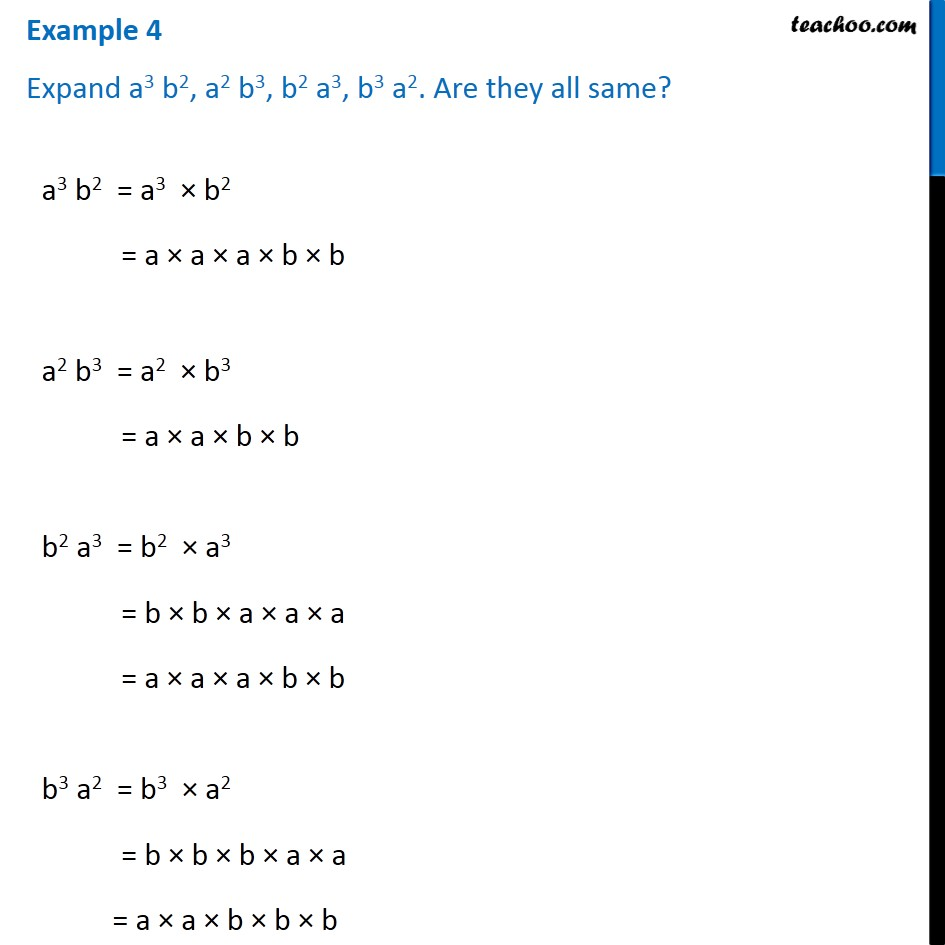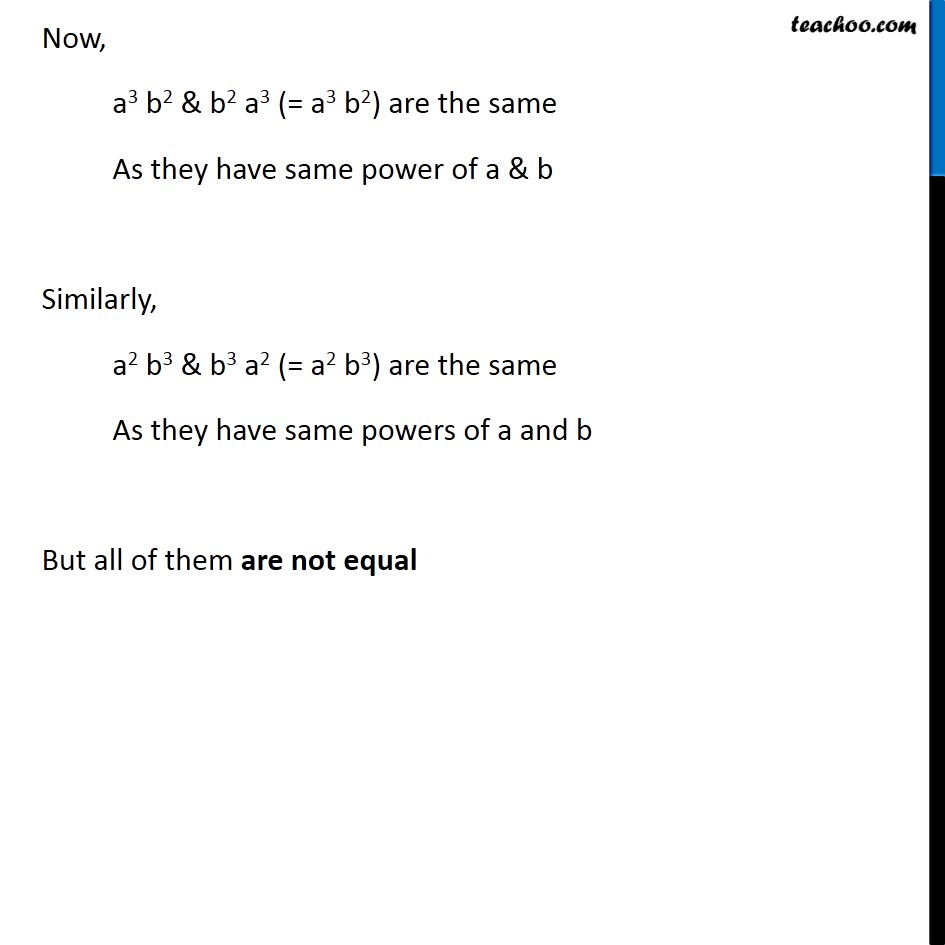Examples

Chapter 11 Class 7 Exponents and Powers
Serial order wiseLearn in your speed, with individual attention - Teachoo Maths 1-on-1 Class

### Transcript

Example 4 Expand a3 b2, a2 b3, b2 a3, b3 a2. Are they all same? a3 b2 = a3 × b2 = a × a × a × b × b a2 b3 = a2 × b3 = a × a × b × b b2 a3 = b2 × a3 = b × b × a × a × a = a × a × a × b × b b3 a2 = b3 × a2 = b × b × b × a × a = a × a × b × b × b Now, a3 b2 & b2 a3 (= a3 b2) are the same As they have same power of a & b Similarly, a2 b3 & b3 a2 (= a2 b3) are the same As they have same powers of a and b But all of them are not equal